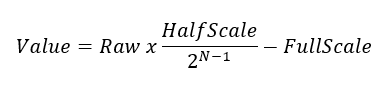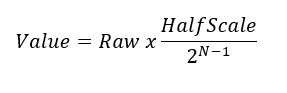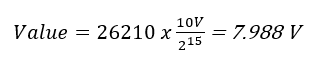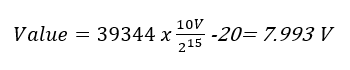## Product FAQs

### How do I convert raw data received through the analog input (AI) channels of an ioLogik E2000 to engineering units?

###### SOLUTION

You can use one of the following formulas to convert raw data (AI channel value; N-bit resolution) to engineering units:

• If the raw data is > the square of 2(N-1), apply the following formula:• If the raw data < the square of 2(N-1), apply the following formula:Example:
If the AI channel value and N-bit resolution are +/-10 V and 16-bit respectively, the FullScale value is 20 V and the HalfScale value is 10 V; square of 2(N-1) is 32768.
• If the raw data is 26210, which is < 32768, the equivalent engineering unit is calculated as follows:• If the raw data is 39344, which is > 32768, the equivalent engineering unit is calculated as follows: